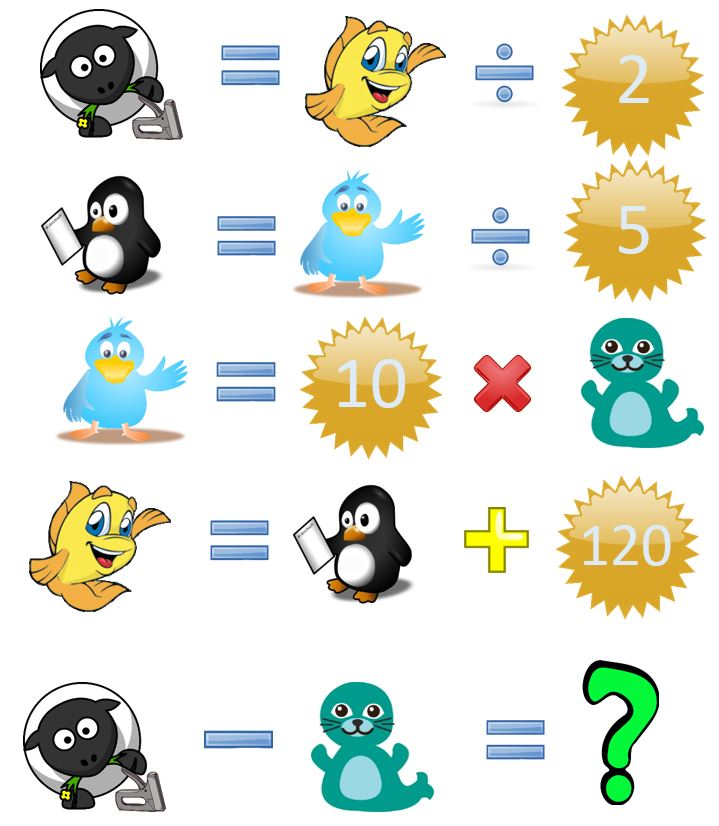# Math Quiz #15Are you able to resolve today’s quiz?
Let’s give it a try 🙂Good luck!:)

If you found the result, just add the answer in the result text below.

## 3 thoughts on “Math Quiz #15”

1.Sunil Kumar sahu says:

Ans= 60

2.Mark Santos says:

60?

3.Cecep Rizal Muhammad Abdullah says:

I think 60

Let see. .
A = D:2 >> 2=D:A >> 2=122:61
So A=61

B = C:5 >> 5= C:B >> 5= 10:2
So B=2 and C=10

C = 10xE >> 10=C:E >> 10=10:1
so E=1

D = B+120 >> 120= D-B >> 120= 122:2 and D=122

A-E = 61-1 =60